# Rank Filters for Image Processing¶

Important: Please read the installation page for details about how to install the toolboxes. $\newcommand{\dotp}{\langle #1, #2 \rangle}$ $\newcommand{\enscond}{\lbrace #1, #2 \rbrace}$ $\newcommand{\pd}{ \frac{ \partial #1}{\partial #2} }$ $\newcommand{\umin}{\underset{#1}{\min}\;}$ $\newcommand{\umax}{\underset{#1}{\max}\;}$ $\newcommand{\umin}{\underset{#1}{\min}\;}$ $\newcommand{\uargmin}{\underset{#1}{argmin}\;}$ $\newcommand{\norm}{\|#1\|}$ $\newcommand{\abs}{\left|#1\right|}$ $\newcommand{\choice}{ \left\{ \begin{array}{l} #1 \end{array} \right. }$ $\newcommand{\pa}{\left(#1\right)}$ $\newcommand{\diag}{{diag}\left( #1 \right)}$ $\newcommand{\qandq}{\quad\text{and}\quad}$ $\newcommand{\qwhereq}{\quad\text{where}\quad}$ $\newcommand{\qifq}{ \quad \text{if} \quad }$ $\newcommand{\qarrq}{ \quad \Longrightarrow \quad }$ $\newcommand{\ZZ}{\mathbb{Z}}$ $\newcommand{\CC}{\mathbb{C}}$ $\newcommand{\RR}{\mathbb{R}}$ $\newcommand{\EE}{\mathbb{E}}$ $\newcommand{\Zz}{\mathcal{Z}}$ $\newcommand{\Ww}{\mathcal{W}}$ $\newcommand{\Vv}{\mathcal{V}}$ $\newcommand{\Nn}{\mathcal{N}}$ $\newcommand{\NN}{\mathcal{N}}$ $\newcommand{\Hh}{\mathcal{H}}$ $\newcommand{\Bb}{\mathcal{B}}$ $\newcommand{\Ee}{\mathcal{E}}$ $\newcommand{\Cc}{\mathcal{C}}$ $\newcommand{\Gg}{\mathcal{G}}$ $\newcommand{\Ss}{\mathcal{S}}$ $\newcommand{\Pp}{\mathcal{P}}$ $\newcommand{\Ff}{\mathcal{F}}$ $\newcommand{\Xx}{\mathcal{X}}$ $\newcommand{\Mm}{\mathcal{M}}$ $\newcommand{\Ii}{\mathcal{I}}$ $\newcommand{\Dd}{\mathcal{D}}$ $\newcommand{\Ll}{\mathcal{L}}$ $\newcommand{\Tt}{\mathcal{T}}$ $\newcommand{\si}{\sigma}$ $\newcommand{\al}{\alpha}$ $\newcommand{\la}{\lambda}$ $\newcommand{\ga}{\gamma}$ $\newcommand{\Ga}{\Gamma}$ $\newcommand{\La}{\Lambda}$ $\newcommand{\si}{\sigma}$ $\newcommand{\Si}{\Sigma}$ $\newcommand{\be}{\beta}$ $\newcommand{\de}{\delta}$ $\newcommand{\De}{\Delta}$ $\newcommand{\phi}{\varphi}$ $\newcommand{\th}{\theta}$ $\newcommand{\om}{\omega}$ $\newcommand{\Om}{\Omega}$

This numerical tour explores non-linear local filters that proceeds by ordering the pixels in a neighboorhood and selecting a given ranked entry.

In :
from __future__ import division

import numpy as np
import scipy as scp
import pylab as pyl
import matplotlib.pyplot as plt

from nt_toolbox.general import *
from nt_toolbox.signal import *

import warnings
warnings.filterwarnings('ignore')

%matplotlib inline


## Continuous Rank Filtering¶

We consider an image $f : [0,1]^2 \rightarrow \RR$.

For any $\beta \in [0,1]$, we define the rank filter $\phi_\be^B$ of order $\beta$ associated to a set $B$ to be $$g = \phi_\beta^B(f) \qwhereq g(x) = \inf \: \enscond{t \in \RR}{ \mu( f^{-1}(]-\infty,t]) \cap x+B ) \geq \mu(B)/2 }.$$ where $\mu$ is the Lebesgue measure on $\RR$.

One usually assumes that $B$ is the ball of radius $\epsilon>0$ $$B = B_\epsilon = \enscond{x}{\norm{x} \leq \epsilon}.$$

When $\be=0$ (resp. $\be=1$, resp. $\be=1/2$), then $g(x)$ is the miniminimum (resp. maximum, resp. median) value of $f$ in a small neighboorhood of radius $\epsilon$ $$\phi_0^{B_\epsilon}(f)(x) = \umin{\norm{y-x} \leq \epsilon} f(y),$$ $$\phi_{1/2}^{B_\epsilon}(f)(x) = \umax{\norm{y-x} \leq \epsilon} f(y),$$ $$\phi_{1}^{B_\epsilon}(f)(x) = \underset{\norm{y-x} \leq \epsilon}{\text{median}} f(y).$$

The operator $\phi_\beta^B$ is contrast-invariant, meaning that it computes with increasing functions $\psi : \RR \rightarrow \RR$ $$\phi_\beta^B \circ \psi = \psi \circ \phi_\beta^B.$$ The axiomatic study of contrast invariant operator was initiated in the comunity of mathematical morphology, see Matheron75, Tukey77, Serra82.

Note also that there exist generalization of rank filters (and in particular the median filter) to vector valued images $f : [0,1]^2 \rightarrow \RR^d$. Since the notion of rank does not exists anymore, one has to rely on variational caracteriation of the median, see for instance CasSapChu00.

The medial filtering is the most popular rank filter. It is particularly efficient to remove impulse noise, see for instance Piterbarg84, FanHall94. See also AriasDon99 for a theoritical analysis of median filtering and of a two-stage iterated version.

## Patches in Images¶

We apply rank filters to discretized images by interpreting them as piecewise constant functions.

Size $N = n \times n$ of the image.

In :
n = 256


We load an image $f_0 \in \RR^N$.

In :
f0 = load_image("nt_toolbox/data/hibiscus.bmp",n)


Display $f_0$.

In :
plt.figure(figsize = (5,5))
imageplot(f0)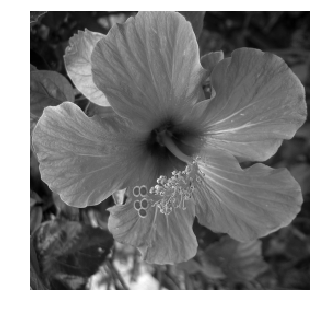Noise level $\si$.

In :
sigma = .04


Generate a noisy image $f=f_0+\epsilon$ where $\epsilon \times \Nn(0,\si^2\text{Id}_N)$.

In :
from numpy import random
f = f0 + sigma*random.standard_normal((n,n))


Display $f$.

In :
plt.figure(figsize = (5,5))
imageplot(clamp(f))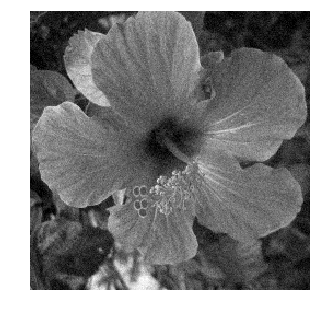For simplicity, we consider the case where the set $B$ is a square of $w_1 \times w_2$ pixels. where we denote $w$ to be the half width of the patches, and $w_1=2w+1$ the full width.

In :
w = 3
w1 = 2*w + 1


We define the patch extraction operator $$p = p_x(f) \in \RR^{w_1 \times w_1} \qwhereq \forall -w \leq s_1,s_2 \leq w, \quad p(s) = f(x+s).$$

We now define the function $\Pi(f) = (p_x(f))_x$ that extracts all possible patches.

We set up large $(n,n,w_1,w_1)$ matrices to index the the X and Y position of the pixel to extract.

In :
[X,Y,dX,dY] = np.meshgrid(np.arange(1,n+1),np.arange(1,n+1),np.arange(-w,w+1),np.arange(-w,w+1))
X = X + dX
Y = Y + dY


We handle boundary condition by reflexion.

In :
X[X < 1] = 2-X[X < 1]
Y[Y < 1] = 2-Y[Y < 1]
X[X > n] = 2*n-X[X > n]
Y[Y > n] = 2*n-Y[Y > n]


Patch extractor operator $\Pi$.

In :
I = (X-1) + (Y-1)*n
for i in range(n//w):
for j in range(n//w):
I[i,j] = np.transpose(I[i,j])

Pi = lambda f: np.reshape(np.ravel(f)[I],(n,n,w1*w1))


We store the patches $\Pi(f)$ as a $n \times n \times w_1^2$ matrix $P$ such that, for each pixel $x$, $P(x)$ is a vector of size $w_1^2$ storing the entries of $p_x(f)$.

In :
P = Pi(f)


Display some example of patches.

In :
from numpy import random

plt.figure(figsize = (5,5))

for i in range(16):
x = random.randint(n)
y = random.randint(n)
imageplot(np.reshape(P[x, y],(w1,w1)), '', [4, 4, i+1])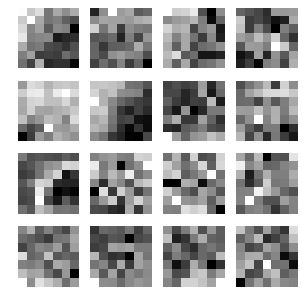## Linear Filter¶

A linear filter (convolution) can be computed using this patch representation as $$g(x) = \sum_{i} \la_i p_x(f)_i.$$

In the case where $\la_i=1/w_1^2$, this defines the mean value inside the patch: $$g(x) = \frac{1}{w_1^2} \sum_{i} p_x(f)_i.$$

In :
Pmean = lambda f: np.mean(Pi(f), 2)


Display it.

In :
plt.figure(figsize = (5,5))
imageplot(Pmean(f))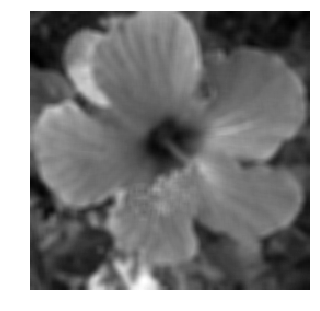Note that this is not a rank filter (this a linear filter) and that it is not contrast invariant. This is shown by displaying $$\phi_\beta^B(f) - \psi^{-1} \circ \phi_\beta^B \circ \psi(f)$$ which is non-zero.

In :
p = 100
psi = lambda f: f**(1/p)
ipsi = lambda f: f**p

plt.figure(figsize = (5,5))
imageplot(Pmean(abs(f)) - ipsi(Pmean(psi(abs(f)))))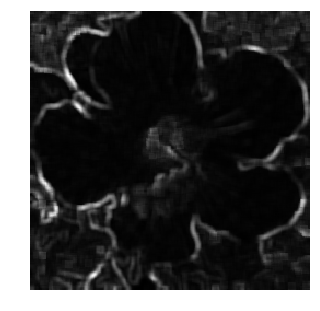## Opening and Closing Rank Filters¶

We now come back to the discrete computation of a rank filter $\phi_\be^B$ for $B$ a square of width $w_1 \times w_1$ pixels.

It is defined as $g=\phi_\beta^B(f)$ where $$g(x) = \text{rank}_{r(\beta)}( p_x(f) )$$ where $\text{rank}_r(v)$ extracted the element of order $k$ in the sorted value of $v \in \RR^Q$ (here $Q=w_1^2$). More precisely, we denote $$v_{\si(1)} \leq v_{\si(2)} \leq \ldots \leq v_{\si(Q)}$$ where $\si \in \Sigma_Q$ is an ordering permutation, which can be computed in $O(N \log(N))$ operations with the QuickSort algorithm. Then the ranked valued is $$\text{rank}_r(v) = v_{\si(r)}.$$

In order to be consistent with the continuous definition of the rank filter, one should define the rank as $$r=r(\beta) = \lfloor Q r \rfloor.$$

In :
r = lambda beta: min(np.ceil(beta*w1*w1), w1*w1-1)


Shortcut for the rank filter.

In :
subsample = lambda x,s : x[: , : , s]
phi = lambda f, beta: subsample(np.sort(Pi(f), 2), r(beta))


Exercise 1

Compute the rank filter for several values of $\beta$.

In :
run -i nt_solutions/denoisingadv_7_rankfilters/exo1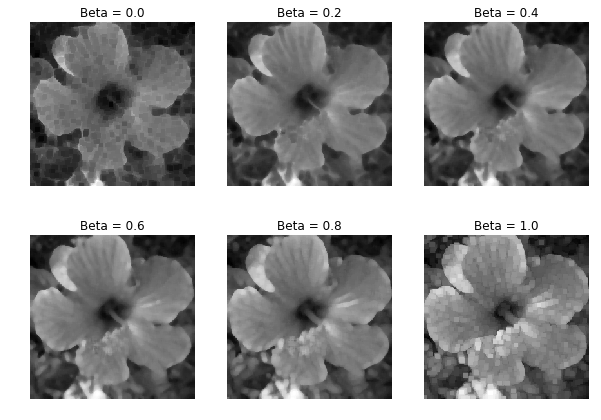In :
## Insert your code here.


The case $\beta=0$ corresponds to the closing operator from mathematical morphology (min filter).

In :
closing = lambda f: phi(f, 0)
plt.figure(figsize = (5,5))
imageplot(closing(f))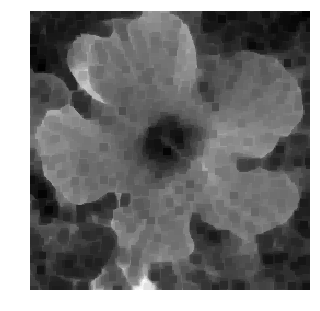The case $\beta=1$ corresponds to the opening operator from mathematical morphology (max filter).

In :
opening = lambda f: phi(f, 1)
plt.figure(figsize = (5,5))
imageplot(opening(f))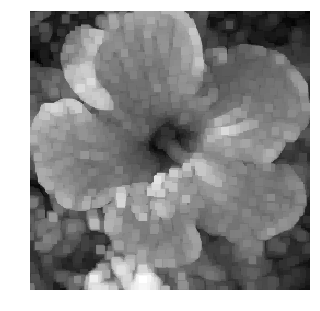Exercise 2

Compute a closing followed by an opening.

In :
run -i nt_solutions/denoisingadv_7_rankfilters/exo2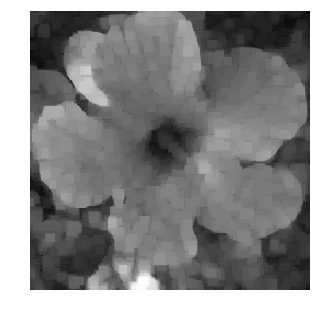In :
## Insert your code here.


Exercise 3

Compute an opening followed by a closing.

In :
run -i nt_solutions/denoisingadv_7_rankfilters/exo3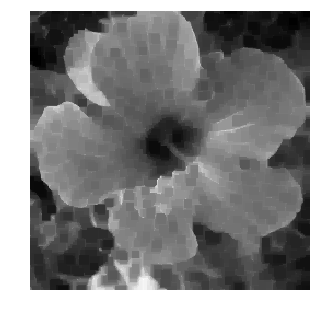In :
## Insert your code here.


Exercise 4

Perform iterated opening and closing.

In :
run -i nt_solutions/denoisingadv_7_rankfilters/exo4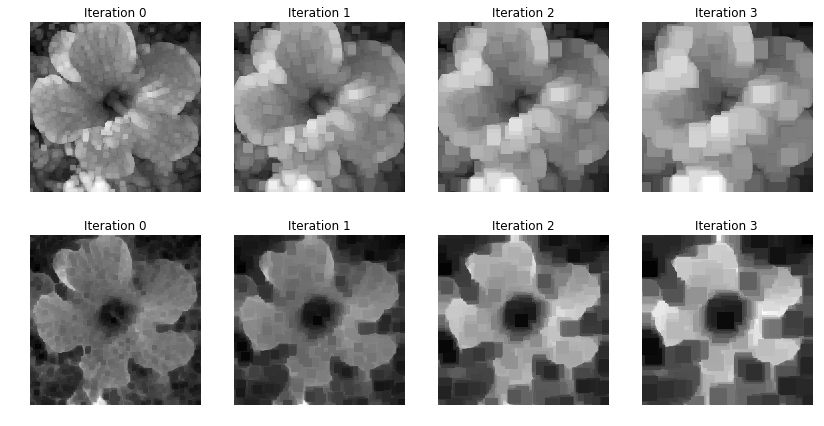In :
## Insert your code here.


## Median Filter¶

The median filter corresponds to the case where $\be=1/2$.

In :
medfilt = lambda f: phi(f, 1/2)


Display the result.

In :
plt.figure(figsize = (5,5))
imageplot(medfilt(f))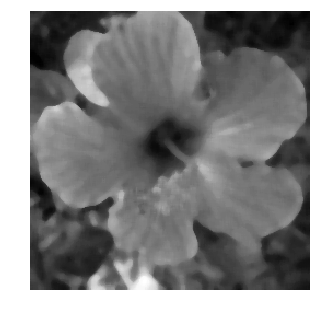Iterated median filtering computes $$f^{(\ell+1)} = \phi_{1/2}^B( f^{(\ell)} ).$$

In the case where $f$ is of class $C^3$ and $\nabla f(x) \neq 0$, one has the following Taylor expansion $$\phi_{1/2}^{B_\epsilon}(x) = f(x) + \frac{\epsilon^2}{6} \norm{\nabla f(x)} \text{Curv}(f)(x) + O(\epsilon^{7/3})$$ where the curvature operator is $$\text{Curv}(f) = \text{div}\pa{ \frac{\nabla f}{\norm{\nabla f}} }.$$

Intuitively, it means that if one iterates the operator $\phi_{1/2}^{B_\epsilon}$ with a proper re-scaling $\ell \leftrightarrow t$ and when $\epsilon \rightarrow 0$, then $f^{(\ell)}$ tends to the solution to the famous mean-curvature motion PDE $$\pd{f}{t} = \norm{\nabla f} \text{Curv}(f).$$

This conjecture was initially mentionned in BeMerOsh92. This was rigorously proved in Ishii95, BarGeorg, Evans93 using the machinery of viscosity solutions.

Similar result holds for other class of contrast invariant operator, see for instance Cao98 for affine invariant operators, and GuiMoRy04 for an axiomatic and general framework.

Exercise 5

Perform iterated median filtering, and store the output in $f_1$.

In :
run -i nt_solutions/denoisingadv_7_rankfilters/exo5